###### Measure the correlation between numerical and categorical variables and the correlation between two categorical variables in Python: Chi-Square and ANOVA
2020-08-02###### Double DQN and Dueling DQN in Reinforcement Learning
2020-09-10

Gradient boost is a machine learning algorithm that works on the ensemble technique called ‘Boosting’. Like other boosting models, Gradient boost sequentially combines many weak learners to form a strong learner. Typically Gradient boost uses decision trees as weak learners. Gradient boost is one of the most powerful techniques for building predictive models for both classification and Regression problems.

### What is Boosting?

Boosting idea is to train weak learners sequentially, each trying to correct its predecessor. This means, the algorithm is always going to learn something which is not completely accurate but a small step in the right direction. As the algorithm moves forward by sequentially correcting the previous errors, it improves the prediction power.

Gradient Boosting can be compared to AdaBoost, but has a few differences :

• Instead of growing a forest of stumps, we initially predict the average (since it’s regression here) of the y-column and build a decision tree based on that value.
• Like in AdaBoost, the next tree depends on the error of the previous one.
• But unlike AdaBoost, the tree we grow is not only a stump but a real decision tree.
• As in AdaBoost, there is a weight associated with the trees, but the scale factor is applied to all the trees.

To understand the Gradient boost below is the steps involved. In Gradient boosting weak learners are decision trees.

Step1: Construct a base tree with a single root node. It is the initial guess for all the samples.

Step2: Build a tree from errors of the previous tree.

Step3: Scale the tree by learning rate (value between 0 and 1). This learning rate determines the contribution of the tree to the prediction

Step4: Combine the new tree with all the previous trees to predict the result and repeat step 2 until a maximum number of trees is achieved or until the new trees don’t improve the fit.

The final prediction model is the combination of all the trees.

### Regression Example

To understand how Gradient boost works, let’s go through a simple example.

Suppose we have the below table of sample data with Height, Age, and Gender as input variables and weight as the output variable.

To predict the weights, step 1 is to create a tree with the root node. For the initial guess, we can use average, mean squared error, mean absolute error, etc.,

If we assume that the average of weights of all the samples is our initial guess then 71.2 (88+76+56+73+77+57/6=71.2) would be our initial root node.

Step 2 is to build a tree based on errors from the previous tree. The error that the previous tree made is the difference between the Actual weight and the predicted weight. This difference is called Pseudo Residual.

Now we build a tree with maximum leaf nodes of 4 using Height, Age, and Gender to predict the residuals(Error). If more than 1 weight falls on the same leaf, then we take the average of the weights as the leaf node.

Step 3 is scaling tree with a learning rate. Assuming the learning rate as 0.1.

Step 4 is combining the trees to make the new prediction. So, we start with initial prediction 71.2 and run the sample data down the new tree and sum them.

If we observe the new predicted weights, we can see a small improvement in the result compared to the average weight from the initial assumption. To further improve the result, we repeat steps 2 and 3 and build another tree from the new pseudo residuals to predict the weights.

Again build a new tree with the new pseudo residuals.

Now we combine the new tree with all the previous trees to predict the new weights. So, we start with the initial prediction and sum it with the scaled result of 1st tree and then sum it with the scaled result of the new tree.

From the new predicted weight, we can observe there is further improvement in the result. Again we calculate the pseudo weights and build a new tree in a similar way. These steps are repeated several times until the new tree doesn’t decrease the pseudo residual value or till a maximum number of trees is built.

So the final predicted model would be

Now if we get new data for the test, we pass it through the above model and calculate the weight of the person.

## Delve into mathematics

Let’s consider a simple scenario in which we have several features, x1,x2,x3,x4, and try to predict y.

Step 1: Make the first guess

The initial guess of the Gradient Boosting algorithm is to predict the average value of the target y. For example, if our features are the age x1 and the height x2 of a person… and we want to predict the weight of the person.

Step 2: Compute the pseudo-residuals

For each sample, we compute the difference between the observations and the prediction we made. These are called pseudo-residuals.

We compute the pseudo-residuals for the first feature x1.

Step 3: Predict the pseudo-residuals

Then, we will be using the features x1,x2,x3, and x4 to predict the pseudo-residuals column.

We can now predict the pseudo-residuals using a tree, that typically has 8 to 32 leaves (so larger than a stump). By restricting the number of leaves of the tree we build, we obtain fewer leaves than residuals. Therefore, the outcome of a given branch of the tree is the average of the columns that lead to this leaf, as in a regression tree.

Step 4: Make a prediction and compute the residuals

To make a prediction, we say that the average is 13.39. Then, we take our observation, run it through the tree, get the value of the leaf, and add it to 13.39.

If we stop here, we will most probably overfit. Gradient Boost applies a learning rate lr to scale the contribution from a new tree, by applying a factor between 0 and 1.The idea behind the learning rate is to make a small step in the right direction. This allows an overall lower variance.

Notice how all the residuals got smaller now.

Step 5: Make a second prediction

Now, we :

• build a second tree
• compute the prediction using this second tree
• compute the residuals according to the prediction
• build the third tree

Let’s just cover how to compute the prediction. We are still using the features x1,x2,x3, and x4 to predict the new residuals Pseudo_Res_2.

We build a tree to estimate those residuals. Once we have this tree (with a limited number of leaves), we are ready to make the new prediction:The prediction is equal to :

• the average value initially computed
• plus LR * the predicted residuals at step 1
• plus LR * the predicted residuals at step 2

Notice how we always apply the same Learning Rate. We are now ready to compute the new residuals, fit the 3rd tree on it, compute the 4th residuals… and so on, until :

• we reach the maximum number of trees specified
• or we don’t learn significantly anymore

### Full Pseudo-code

The algorithm can be then described as the following, on a dataset (x,y) with x the features and y the targets, with a differentiable loss function: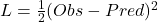called the Squared Residuals. Notice that since the function is differentiable, we have:Step 1: Initialize the model with a constant value:We simply want to minimize the sum of the squared residuals (SSR) by choosing the best prediction.

If we derive the optimal value for: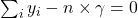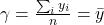This is simply the average of the observations. This justifies our previous constant initialization. In other words, we created a leaf that predicts all samples will weigh the average of the samples.

Step 2: For m = 1 to M (the maximum number of trees specified, e.g 100)

• a) Compute the pseudo-residuals for every sample :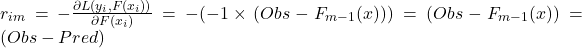This derivative is called the Gradient. The Gradient Boost is named after this.

• b) Fit a regression tree to the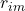values and create terminal regions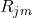for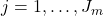, i.e create the leaves of the tree. At that point, we still need to compute the output value of each leaf.
• c) For each leaf, compute the output value that minimized the SSR:. In other words, we will simply predict the output of all the samples stored in a certain leaf.
• d) Make a new prediction for each sample by updating, accoridng to a learning rate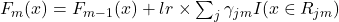. We compute the new value by summing the previous prediction and all the predictions γγ into which our sample falls.

## Implement a high-level Gradient Boosting in Python

Since the pseudo-code detailed above might be a bit tricky to understand, I’ve tried to summarize a high-level idea of Gradient Boosting, and we’ll be implementing it in Python.

Step 1: Initialize the model with a constant value:This is simply the average of the observations.

Step 2: For each tree m = 1 to M (the maximum number of trees specified, e.g 100)

• a) Compute the pseudo-residuals for every sample, i.e the true value – the predicted value :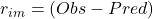• b) Fit a regression tree on the residuals, and predict the residuals• c) Update the prediction :### Data generation

We start by generating some data for our regression :

import numpy as np
import pandas as pd
import matplotlib.pyplot as plt

x = np.arange(0,50)
x = pd.DataFrame({'x':x})

y1 = np.random.uniform(10,15,10)
y2 = np.random.uniform(20,25,10)
y3 = np.random.uniform(0,5,10)
y4 = np.random.uniform(30,32,10)
y5 = np.random.uniform(13,17,10)

y = np.concatenate((y1,y2,y3,y4,y5))
y = y[:,None]

plt.figure(figsize=(12,8))
plt.scatter(x,y)
plt.show()


## Fit a simple decision tree

To illustrate the limits of decision trees, we can try to fit a simple decision tree with a maximal depth of 1, called a stump.

from sklearn import tree

clf = tree.DecisionTreeRegressor(max_depth=1)
model = clf.fit(x,y)
pred = model.predict(x)

plt.figure(figsize=(12,8))
plt.plot(x, pred, c='red')
plt.scatter(x,y)
plt.show()


This is the starting point for our estimation. Now, we need to go further and make our model more complex by implementing gradient boosting.

xi = x.copy()
yi = y.copy()

# Initialize error to 0
ei = 0
n = len(yi)

# Initialize predictions with average
predf = np.ones(n) * np.mean(yi)

lr = 0.3

# Iterate according to the number of iterations chosen
for i in range(101):

# Step 2.a)
# Fit the decision tree / stump (max_depth = 1) on xi, yi

clf = tree.DecisionTreeRegressor(max_depth=1)
model = clf.fit(xi, yi)

# Use the fitted model to predict yi

predi = model.predict(xi)

# Step 2.c)
# Compute the new prediction (learning rate !)
# Compute the new residuals,
# Set the new yi equal to the residuals

predf = predf + lr * predi
ei = y.reshape(-1,) - predf
yi = ei

# Every 10 iterations, plot the prediction vs the actual data
if i % 10 == 0 :
plt.figure(figsize=(12,8))
plt.plot(x, predf, c='r')
plt.scatter(x, y)
plt.title("Iteration " + str(i))
plt.show()


By increasing the learning rate, we tend to overfit. However, if the learning rate is too low, it takes a large number of iterations to even approach the underlying structure of the data.

## Conclusion

Gradient boost is a powerful boosting technique. It improves the accuracy of the model by sequentially combining weak trees to form a strong tree. In this way, it achieves low bias and low variance. I hope this introduction to Gradient Boosting was helpful. The topic can get much more complex over time, and the implementation is Scikit-learn is much more complex than this.

References:

https://www.numpyninja.com/blog